# RS Aggarwal Solutions Class 10 Chapter 3 - Linear Equations In Two Variables

## RS Aggarwal Class 10 Chapter 3 Linear Equations In Two Variables Solutions Free PDF

A linear equation in two variables contains two different coefficients along with two variables. Linear equations are primarily represented in two forms which are basically Cartesian coordinates, Slope-Intercept form, Intercept form, Two-point form, Matrix form and Parametric form. The linear equation which is usually written as y= f(x), can be additivity and homogeneity. The general format of a linear equation in two variables can be represented as:

ax+ by + c= 0

The solutions provided here for Class 10 Chapter 3 of all the exercises can be used when you have any confusion while solving the RS Aggarwal Class 10 Solutions. The solutions are given with step-wise explanation and in detail to make learning easy for students.  Solving the exercise questions will help students to perform well in their final exam and also they can self-evaluate their performance level. It is a perfect revision study material as it is prepared as per the CBSE Class 10 Maths syllabus.

## Download PDF of RS Aggarwal Solutions Class 10 Chapter 3- Linear Equations in Two Variables

Learn more about RS Aggarwal Class 10 Solutions Chapter 3 Linear equation in two variables, available below:

Question 1: On a graph paper, draw a horizontal line X’OX and a vertical line YOY’ as the x-axis and the y-axis respectively.

2x + 3y = 2, x – 2y = 8

Solution:

Given equations are 2x + 3y = 2 and x – 2y = 8

Graph of 2x + 3y = 2:

$y = \frac{2(1 – x)}{3}$

Putting x = 1, we get y = 0

Putting x = -2, we get y = 2

Putting x = 4, we get y = -2

Hence, the table is:

 x 1 -2 4 y 0 2 -2

Plot the points A (1, 0), B (-2, 2) and C (4, -2) on the graph paper. Join AB and AC to get the graph line BC. Extend it both ways.

Thus, line BC is the graph of 2x + 3y = 2.

Graph of x – 2y = 8:

$y = \frac{x – 8}{2}$

Putting x = 2, we get y = -3

Putting x = 4, we get y = -2

Putting x = 0, we get y = -4

Hence, the table is:

 x 2 4 0 y -3 -2 -4

Now, on the same graph paper as above plot the points P (0, -4) and Q (2, -3). The point C (4, -2) has already been plotted. Join QC and extend it. Thus, the line PC is the graph of x – 2y = 8.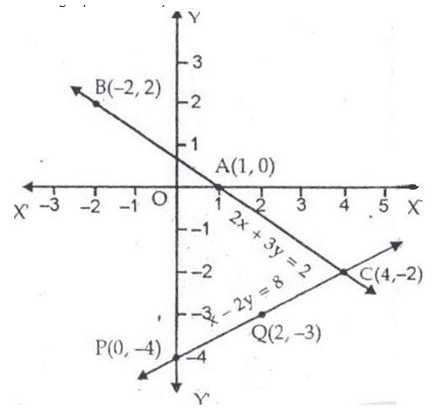The two graph lines intersect at C (4, -2). Therefore, x = 4, y = -2 is the solution of given system of equations.

Question 2: On a graph paper, draw a horizontal line X’OX and a vertical line YoY’ as the x-axis and the y-axis respectively.

3x + 2y = 4 and 2x – 3y = 7

Solution:

Given equations are 3x + 2y = 4 and 2x – 3y = 7

Graph of 3x + 2y = 4:

$y = \frac{4 – 3x}{2}$

Putting x = 0, we get y = 2

Putting x = 2, we get y = -1

Putting x = -2, we get y = 5

Hence, the table is:

 x 0 2 -2 y 2 -1 5

Plot the points A (0, 2), B (2, -1) and C (-2, 5) on the graph paper. Join AB and AC to get the graph line BC. Extend it both ways.

Thus, line BC is the graph of 3x + 2y = 4.

Graph of 2x – 3y = 7:

$y = \frac{x – 8}{2}$

Putting x = 2, we get y = -1

Putting x = -1, we get y = -3

Putting x = 5, we get y = 1

Hence, the table is:

 x 2 -1 5 y -1 -3 1

Now, on the same graph paper as above plot the points P (-1, -3) and Q (5, 1). The point B (2, -1) has already been plotted. Join QB and extend it. Thus, the line PQ is the graph of 2x – 3y = 7.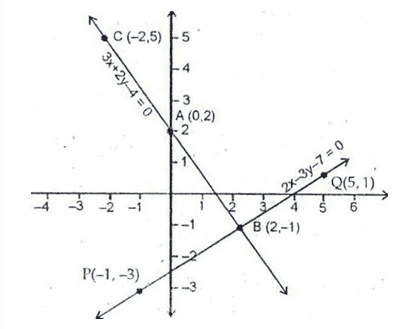The two graph lines intersect at B (2,-1). Therefore, x = 2, y = -1 is the solution of given system of equations.

Question 3: On a graph paper, draw a horizontal line X’OX and a vertical line YoY’ as the x-axis and the y-axis respectively.

x – y + 1 = 0,

3x + 2y – 12 = 0

Solution:

Given equations are x – y + 1 = 0 and 3x + 2y – 12 = 0

Graph of x – y + 1 = 0:

x – y + 1 = 0 $\Rightarrow$ y = x + 1 ……..(1)

Putting x = 0, we get y = 1

Putting x = -1, we get y = 0

Putting x = 2, we get y = 3

Hence, the table is:

 x 0 -1 2 y 1 0 3

Plot the points A (0, 1), B (-1, 0) and C (2, 3) on the graph paper. Join AB and AC to get the graph line BC. Extend it both ways.

Thus, line BC is the graph of x – y + 1 = 0.

Graph of 3x + 2y – 12 = 0:

$y = \frac{12 – 3x}{2}$

Putting x = 0, we get y = 6

Putting x = 2, we get y = 3

Putting x = 4, we get y = 0

Hence, the table is:

 x 0 2 4 y 6 3 0

Now, on the same graph paper as above plot the points P (0, 6) and Q (4, 0). The point C (2, 3) has already been plotted. Join QB and extend it. Thus, the line PQ is the graph of 3x + 2y – 12 = 0.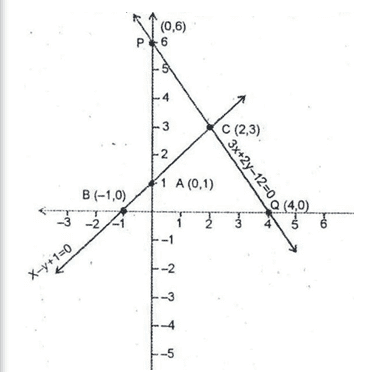The two graph lines intersect at (2, 3). Therefore, x = 2, y = 3 is the solution of given system of equations.

Question 4: On a graph paper, draw a horizontal line X’OX and a vertical line YoY’ as the x-axis and the y-axis respectively.

2x + 3y = 4, 3x – y = -5

Solution:

Given equations are 2x + 3y = 4 and 3x – y = -5

Graph of 2x + 3y = 4:

2x + 3y = 4 $\Rightarrow y = \frac{4 – 2x}{3}$

Putting x = -1, we get y = 2

Putting x = 2, we get y = 0

Putting x = 5, we get y = -2

Hence, the table is:

 x -1 2 5 y 2 0 -2

Plot the points A (-1, 2), B (2, 0) and C (5, -2) on the graph paper. Join AB and BC to get the graph line AC. Extend it both ways.

Thus, line BC is the graph of 2x + 3y = 4.

Graph of 3x – y = -5:

y = 3x + 5

Putting x = -1, we get y = -2

Putting x = 0, we get y = 5

Putting x = -2, we get y = -1

Hence, the table is:

 x -1 0 -2 y 2 5 -1

Now, on the same graph paper as above plot the points P(0, 5) and Q(-2, -1). The point A (-1, 2) has already been plotted. Join PA and amd QA to get line PQ. Thus, the line PQ is the graph of 3x – y = -5.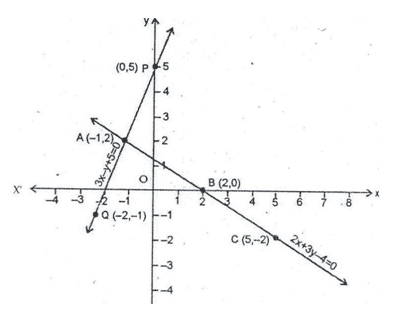The two graph lines intersect at A (-1, 2). Therefore, x = -1, y = 2 is the solution of given system of equations.

Question 5: On a graph paper, draw a horizontal line X’OX and a vertical line YoY’ as the x-axis and the y-axis respectively.

2x – 3y = 1, 3x – 4y = 1

Solution:

Given equations are 2x – 3y = 1 and 3x – 4y = 1

Graph of 2x – 3y = 1:

2x – 3y = 1 $\Rightarrow y = \frac{2x – 1}{3}$

Putting x = -1, we get y = -1

Putting x = 2, we get y = 1

Putting x = 5, we get y = 3

Hence, the table is:

 x -1 2 5 y -1 1 3

Plot the points A (-1, -1), B (2, 1) and C (5, 3) on the graph paper. Join AB and BC to get the graph line AC. Extend it both ways.

Thus, line AC is the graph of 2x – 3y = 1.

Graph of 3x – 4y = 1:

3x – 4y = 1 $\Rightarrow y = \frac{3x – 1}{4}$

Putting x = -1, we get y = -1

Putting x = 3, we get y = 2

Putting x = 2, we get y = -4

Hence, the table is:

 x -1 3 -5 y -1 2 -4

Now, on the same graph paper as above plot the points P (3, 2) and Q(-5, -4). The point A (-1, -1) has already been plotted. Join PA and QA. Thus, the line PQ is the graph of 3x – 4y = 1.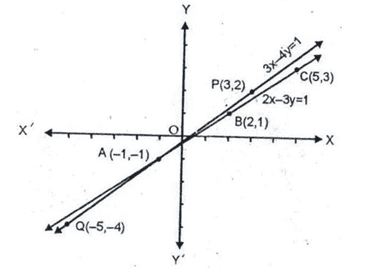The two graph lines intersect at A (-1, -1). Therefore, x = -1, y = -1 is the solution of given system of equations.

Question 6: On a graph paper, draw a horizontal line X’OX and a vertical line YoY’ as the x-axis and the y-axis respectively.

4x + 3y = 5, 2y – x = 7

Solution:

Given equations are 4x + 3y = 5 and 2y – x = 7

Graph of 4x + 3y = 5:

4x + 3y = 5 $\Rightarrow y = \frac{5 – 4x}{3}$

Putting x = -1, we get y = 3

Putting x = 2, we get y = -1

Putting x = 5, we get y = -5

Hence, the table is:

 x -1 2 5 y 3 -1 -5

Plot the points A (-1, 3), B (2, -1) and C (5, -5) on the graph paper. Join AB and BC to get the graph line AC. Extend it both ways.

Thus, line AC is the graph of 4x + 3y = 5.

Graph of 2y – x = 7:

2y – x = 7$\Rightarrow y = \frac{7 + x}{2}$

Putting x = -1, we get y = 3

Putting x = 3, we get y = 5

Putting x = -3, we get y = 2

Hence, the table is:

 x -1 3 -3 y 3 5 2

Now, on the same graph paper as above plot the points P (3, 5) and Q (-3, 2). The point A (-1, 3) has already been plotted. Join PA and PQ. Thus, the line PQ is the graph of 2y – x = 7.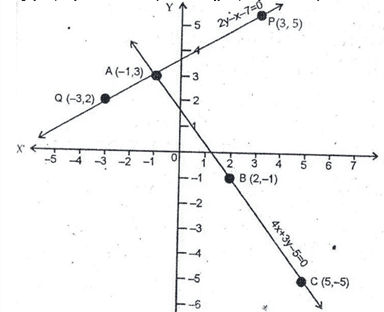The two graph lines intersect at A(-1, -3). Therefore, x = -1, y = 3 is the solution of given system of equations.

Question 7: On a graph paper, draw a horizontal line X’OX and a vertical line YoY’ as the x-axis and the y-axis respectively.

x + 2y + 2 = 0, 3x + 2y + 2 = 0

Solution:

Given equations are x + 2y + 2 = 0 and 3x + 2y + 2 = 0

Graph of x + 2y + 2 = 0:

x + 2y + 2 = 0 $\Rightarrow y = \frac{-x -2}{2}$

Putting x = -2, we get y = 0

Putting x = 0, we get y = -1

Putting x = 2, we get y = -2

Hence, the table is:

 x -2 0 2 y 0 -1 -2

Plot the points A (-2, 0), B (0, -1) and C (2, -2) on the graph paper. Join AB and BC to get the graph line AC. Extend it both ways.

Thus, line AC is the graph of x + 2y + 2 = 0.

Graph of 3x + 2y + 2 = 0:

3x + 2y + 2 = 0 $\Rightarrow y = \frac{-3x + 2}{2}$

Putting x = 0, we get y = 1

Putting x = 2, we get y = -2

Putting x = 4, we get y = -5

Hence, the table is:

 x 0 2 4 y 1 -2 -5

Now, on the same graph paper as above plot the points P (0, 1) and Q (4, -5). The point C (2, -2) has already been plotted. Join PC and QC. Thus, the line PQ is the graph of 3x + 2y + 2 = 0.

The two graph lines intersect at C(2, -2). Therefore, x = 2, y = -2 is the solution of given system of equations.

Question 8: On a graph paper, draw a horizontal line X’OX and a vertical line YoY’ as the x-axis and the y-axis respectively.

2x + 3y = 8, x – 2y + 3 = 0

Solution:

Given equations are 2x + 3y = 8 and x – 2y + 3 = 0

Graph of 2x + 3y = 8:

2x + 3y = 8 $\Rightarrow y = \frac{8 – 2x}{3}$

Putting x = 1, we get y = 2

Putting x = -5, we get y = 6

Putting x = 7, we get y = -2

Hence, the table is:

 x 1 -5 7 y 2 6 -2

Plot the points A (1, 2), B (-5, 6) and C (7, -2) on the graph paper. Join AB and BC to get the graph line BC. Extend it both ways.

Thus, line AC is the graph of 2x + 3y = 8.

Graph of x – 2y + 3 = 0:

x – 2y + 3 = 0 $\Rightarrow y = \frac{x + 3}{2}$

Putting x = 1, we get y = 2

Putting x = 3, we get y = 3

Putting x = -3, we get y = 0

Hence, the table is:

 x 1 3 -3 y 2 3 0

Now, on the same graph paper as above plot the points P (3, 3) and Q (-3, 0). The point A (1, 2) has already been plotted. Join PA and QA. Thus, the line PQ is the graph of x – 2y + 3 = 0.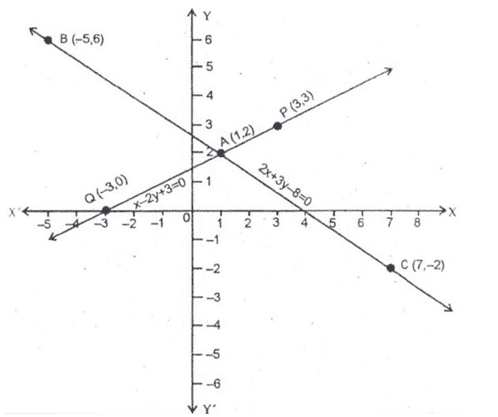The two graph lines intersect at A (1, 2). Therefore, x = 1, y = 2 is the solution of given system of equations.

### RS Aggarwal Solutions Class 10 Chapter 3 – Linear Equations in Two Variables

Students of Class 10 are advised to practice from the RS Aggarwal Class 10 Maths textbook without looking at the solutions, they may look at the solutions if they are unable to solve them. This will help them in developing the confidence to attempt unsolved exercises which is very important if you want to score well in the examination. RS Aggarwal Maths Solutions Class 10 Chapter 3 – Linear Equations in Two Variables is helpful not only because it helps with revisions but also because you can look over the topics quickly.

#### Practise This Question

Silver metal acts as a non-conductor of electricity.